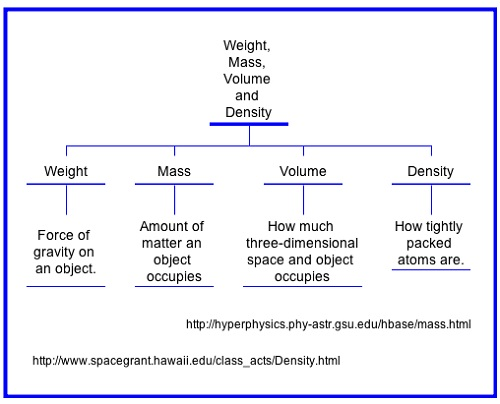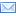#Difference Between Similar Terms and Objects

# Difference Between Density and Weight## Density vs. Weight

Both density and weight are science-related terms mostly used in the field of physics. However, both terms are not exclusive to the realm of science. The term “weight” is also a word used in the health and medical arena, where it holds a different meaning. Additionally, density can be associated with “population density” in the world of statistics and other related fields. Both terms also deal with and are expressed in their own respective mathematical formulas often used in calculations in physics.

“Density” is defined as “the physical property of matter that deals with the mass of an object per volume in itself.” On the other hand, weight is also a physical property of matter that concerns the mass of an object with consideration to the force that affects that object (the force being gravity or lack thereof).

The formula for density is reflected in its definition; density can be derived from the quotient of an object’s mass to its volume, while the formula for weight is mass multiplied by gravity. By looking at the formula of the two concepts, one similar component can be noticed – mass. However, the operations used in the formulas are different; calculating density requires division, whereas calculating weight requires multiplication.

Density deals with volume, which is the product of an object’s height, width, and length. Gravity, which is an important component of the formula for weight, has the complicated formula of F=G ([m1*m2]/D^2), wherein “F” stands for the force of gravity calculated in Newton’s law. “G” represents “R”, the Gravitational Constant (6.673E-11 Newtons). Both M’s stand for the masses of each object, and “D” – for the distance between the centers of the objects.

Furthermore, the terms use different units to represent their respective conditions. In the case of density, this is a compound unit – both the unit of mass and the unit of volume are mentioned. For example, grams per cubic centimeter (g/cm3) or pounds per cubic foot (lb/ft3) are the often-used units for measurement of density. On the other hand, due to gravity’s influence on weight – it, weight is expressed by Newton’s law (symbolized by a capital “N”),.

It is also noted that density has a superscript of 3 after the unit of measurement; this indicates that there are three properties involved in the formula. This is not the case when it comes to weight, which can be expressed in a single unit of measurement.

Another big difference between the two is that density calculates the intrinsic values of its components (mass and volume, which includes height, width, and length), while weight has an outside force as a component (gravity), and mass of the object. Density is also subject to environmental changes such as pressure and temperature of the objects. If the pressure or temperature is modified, density will be affected. Weight is never altered unless the gravity or mass of the object changes.

### Summary:

1.Density has the components of mass and volume, while weight is concerned with mass and gravity.
2.Division is used to find an object’s density by dividing the mass over volume, while weight is the product of mass and gravity (by virtue of multiplication).
3.The units to express density and weight also differ. Newton’s law is used for weight, while the formula for density is the combination of the formulas for mass and volume.
4.Density changes when pressure and temperature of the object change, while in the case of weight, changes only occur when two of its factors (mass and gravity) change.
5.Density deals with four extensive properties of matter: mass, height, length, and width (which constitutes volume), while weight only deals with two – mass and gravity. Gravity is an optional property and only applies when the object is in motion.

Latest posts by Celine (see all)

### Search DifferenceBetween.net :

Custom Search

Help us improve. Rate this post!Loading...Email This Post : If you like this article or our site. Please spread the word. Share it with your friends/family.

### 1 Comment

1. Great Information..